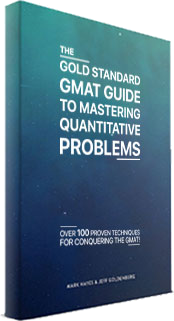to get our Award Winning Self Study GMAT Prep
G
Apr 22, 2016

# 2 GMAT Hacks

One of the trickiest parts about GMAT prep is learning how to balance accuracy and speed. Pure accuracy is rarely fast and pure speed is seldom accurate. Still, you will need both to score high on the GMAT. No GMAT skill better illustrates this than long division. Done correctly, long division allows you to be very accurate, however it is also a slow process. Fortunately there are two hacks that you can use to divide numbers quickly and with enough accuracy to get the answer right. The trick to long division is to not use it at all.

Avoid Long Division

We all learned how to do long division when we were in grade school. When done correctly, long division works just fine and will eventually get you to the answer. The problem is that long division takes a long time. The GMAT is a timed test and you do not have all day to focus on any single question. Additionally, complicated long division involving numbers that are not easily divisible invites a lot of opportunities for mistakes. The good news is that there are several ways to avoid long division. For today, let’s focus on approximation and factoring.

Approximation

The harsh truth is that any time you approximate you reduce the level of accuracy in your answer. However, how accurate is accurate enough to get the answer right? Unfortunately there is no absolute answer to this question. Still, the vast majority of GMAT questions allow some wiggle room. To check how much margin you have, take a look at the answer choices. If all of the choices are within a few tenths or hundredths of each other, you may want to stick with the raw numbers that the question gives you and avoid approximating. However, in my experience it is very rare for all the values to be right on top of each other. This means that in almost all instances you will be able to use rounding and approximation to your advantage.

For example, let’s say that to solve a question you have to divide 10 by 2.487. Using long division to solve for this problem would involve multiple steps and take too much time. Instead, round 2.487 up to 2.5. If you have been practicing your mental math, dividing 10 by 2.5 should be easy and you will arrive at the answer of 4 in no time. Is this answer as accurate as long division? No, but in this example it is only off by two hundredths making it accurate enough to work.

Factoring

Sometimes the GMAT will throw huge numbers at you and ask you to divide them. Don’t fall for the trap and resort to long division. In most cases, you can break the numbers down into more manageable figures by following simple factoring rules. Unless you are dealing with prime numbers, the majority of numbers are a multiple of 2, 3, 4, 5, 6, 9, or 10. The good news is that there are very simple tests to check if any of these numbers are a factor.

Factor of 2: All numbers ending in 2 or 0 can be divided by 2

Factor of 3: If the sum of a number’s digits is divisible by 3, the entire number is too.

Factor of 4: If the last two digits of a number are divisible by 4, the entire number is too.

Factor of 5: All numbers ending in 5 or 0 can be factored by 5.

Factor of 6: If a number is divisible by both 2 and 3 it is also divisible by 6.

Factor of 9: If the sum of a number’s digits is divisible by 9, the entire number is too.

Factor of 10: All numbers ending in 0 can be factored by 10.

So what does all of this look like in practice? Let’s say the GMAT asks you to divide 700 by 25. Solving for this with long division would take an unnecessary length of time. However, by memorizing factoring rules, we know that both numbers are factors of 5. This leaves us with 140 ÷ 5 which is considerably more manageable and we can use mental math to reach the answer of 28.

A large part of the GMAT is learning how to balance accuracy and time. By using these tips to limit the number of times long division has to be used, your GMAT quant scores will rise and your time spent per question will drop.